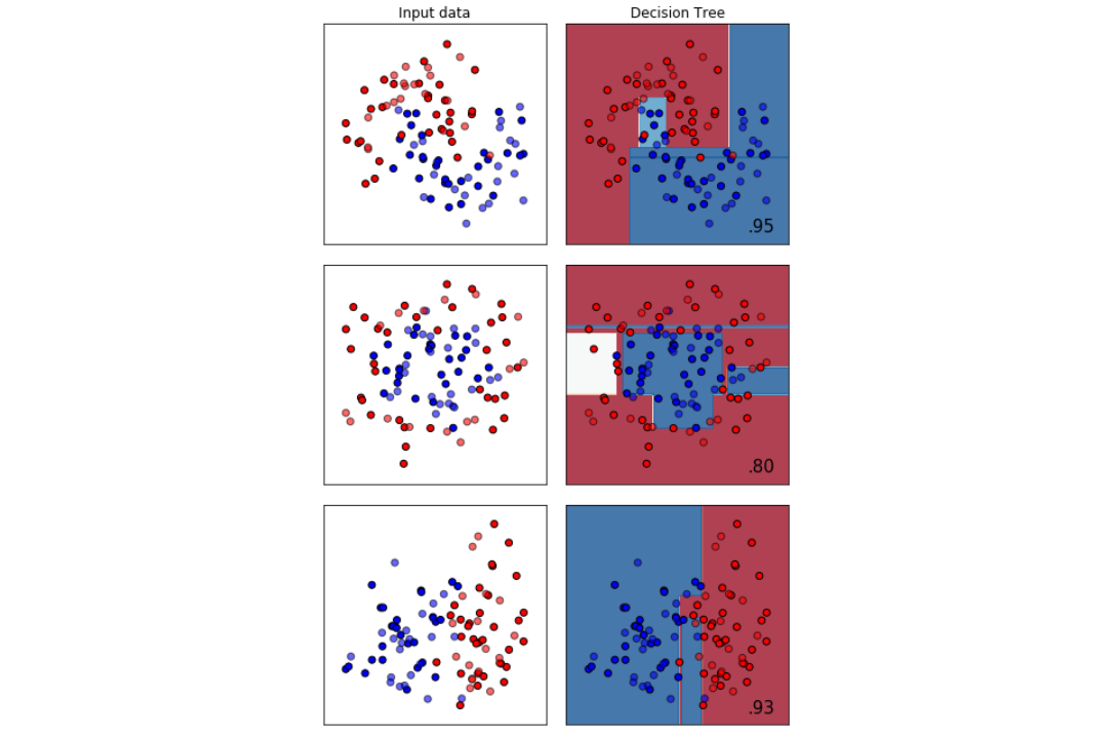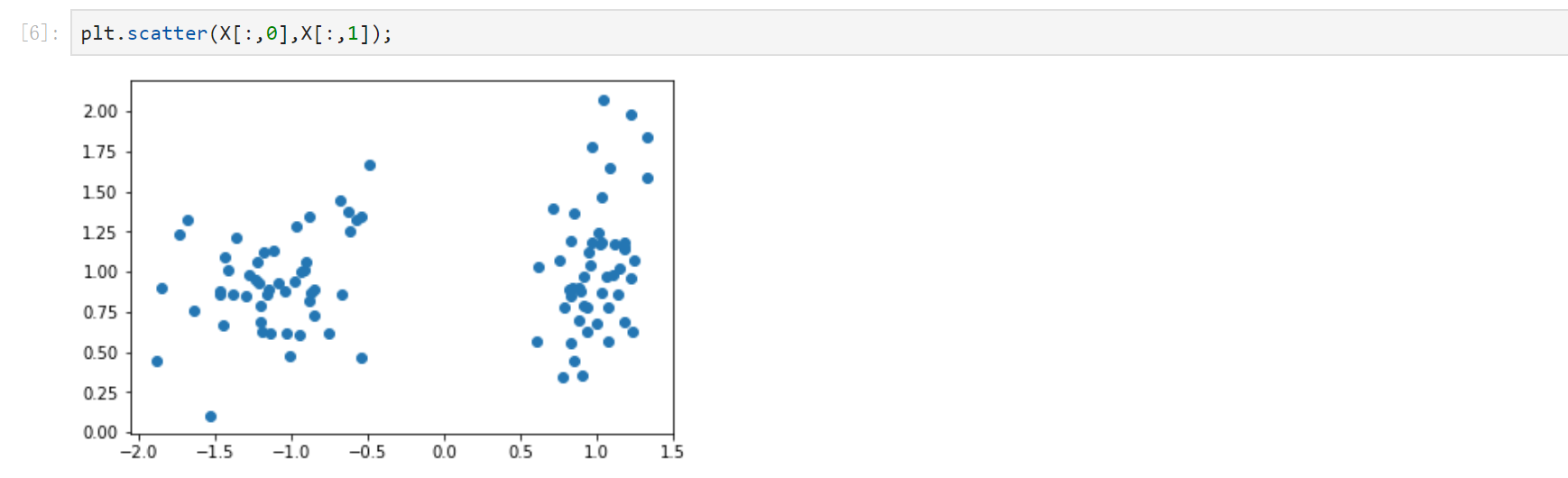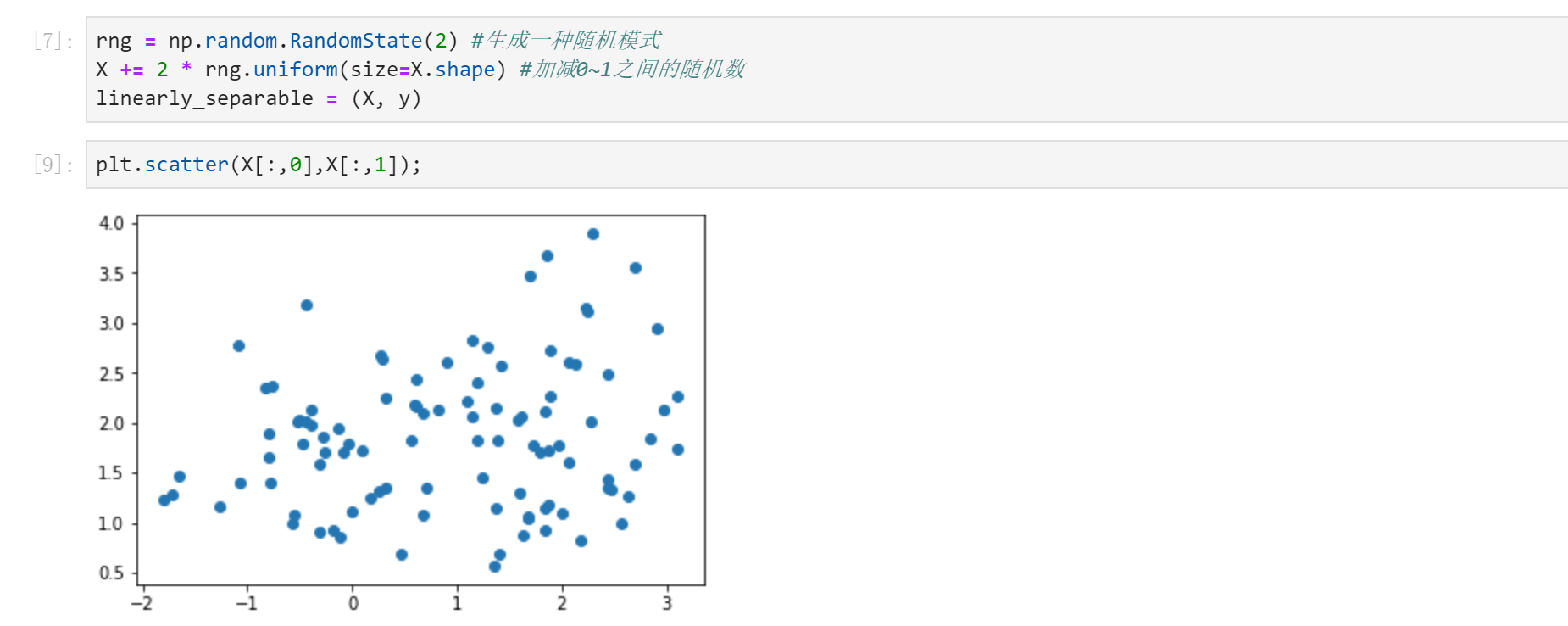## SKLearn分类树在合成数集上的表现

Python 3.7.1（你的版本至少要3.4以上

Scikit-learn 0.20.0 （你的版本至少要0.20

Graphviz 0.8.4 (没有画不出决策树哦，安装代码conda install python-graphviz

Numpy 1.15.3, Pandas 0.23.4, Matplotlib 3.0.1, SciPy 1.1.0### 1. 导入需要的库

``````import numpy as np
import matplotlib.pyplot as plt
from matplotlib.colors import ListedColormap
from sklearn.model_selection import train_test_split
from sklearn.preprocessing import StandardScaler
from sklearn.datasets import make_moons, make_circles, make_classification
from sklearn.tree import DecisionTreeClassifier
``````

### 2. 生成三种数据集

``````#make_classification库生成随机的二分型数据
X, y = make_classification(n_samples=100, #生成100个样本
n_features=2,  #包含2个特征，即生成二维数据
n_redundant=0, #添加冗余特征0个
n_informative=2, #包含信息的特征是2个
random_state=1,  #随机模式1
n_clusters_per_class=1 #每个簇内包含的标签类别有1个
)
``````

``````plt.scatter(X[:,0],X[:,1]);
``````【注意】这个过程只能够运行一次，因为多次运行之后X会变得非常稀疏，两个簇的数据会混合在一起，分类器的效应会继续下降

``````rng = np.random.RandomState(2) #生成一种随机模式
X += 2 * rng.uniform(size=X.shape) #加减0~1之间的随机数
linearly_separable = (X, y)
``````

``````plt.scatter(X[:,0],X[:,1]);
````````````#用make_moons创建月亮型数据，make_circles创建环形数据，并将三组数据打包起来放在列表datasets中
datasets = [make_moons(noise=0.3, random_state=0),
make_circles(noise=0.2, factor=0.5, random_state=1),
linearly_separable]
``````

### 3. 画出三种数据集和三棵决策树的分类效应图像

``````#创建画布，宽高比为6*9
figure = plt.figure(figsize=(6, 9))
#设置用来安排图像显示位置的全局变量i
i = 1

#开始迭代数据，对datasets中的数据进行for循环

for ds_index, ds in enumerate(datasets):

#对X中的数据进行标准化处理，然后分训练集和测试集
X, y = ds
X = StandardScaler().fit_transform(X)
X_train, X_test, y_train, y_test = train_test_split(X, y, test_size=.4, random_state=42)

#找出数据集中两个特征的最大值和最小值，让最大值+0.5，最小值-0.5，创造一个比两个特征的区间本身更大一点的区间
x1_min, x1_max = X[:, 0].min() - .5, X[:, 0].max() + .5
x2_min, x2_max = X[:, 1].min() - .5, X[:, 1].max() + .5

#用特征向量生成网格数据，网格数据，其实就相当于坐标轴上无数个点
#函数np.arange在给定的两个数之间返回均匀间隔的值，0.2为步长
#函数meshgrid用以生成网格数据，能够将两个一维数组生成两个二维矩阵。
#如果第一个数组是narray，维度是n，第二个参数是marray，维度是m。那么生成的第一个二维数组是以narray为行，m行的矩阵，而第二个二维数组是以marray的转置为列，n列的矩阵
#生成的网格数据，是用来绘制决策边界的，因为绘制决策边界的函数contourf要求输入的两个特征都必须是二维的
array1,array2 = np.meshgrid(np.arange(x1_min, x1_max, 0.2),
np.arange(x2_min, x2_max, 0.2))

#接下来生成彩色画布
#用ListedColormap为画布创建颜色，#FF0000正红，#0000FF正蓝
cm = plt.cm.RdBu
cm_bright = ListedColormap(['#FF0000', '#0000FF'])

#在画布上加上一个子图，数据为len(datasets)行，2列，放在位置i上
ax = plt.subplot(len(datasets), 2, i)

#到这里为止，已经生成了0~1之间的坐标系3个了，接下来为我们的坐标系放上标题
#我们有三个坐标系，但我们只需要在第一个坐标系上有标题，因此设定if ds_index==0这个条件
if ds_index == 0:
ax.set_title("Input data")

#将数据集的分布放到我们的坐标系上
#先放训练集
ax.scatter(X_train[:, 0], X_train[:, 1], c=y_train,
cmap=cm_bright,edgecolors='k')
#放测试集
ax.scatter(X_test[:, 0], X_test[:, 1], c=y_test,
cmap=cm_bright, alpha=0.6,edgecolors='k')

#为图设置坐标轴的最大值和最小值，并设定没有坐标轴
ax.set_xlim(array1.min(), array1.max())
ax.set_ylim(array2.min(), array2.max())
ax.set_xticks(())
ax.set_yticks(())

#每次循环之后，改变i的取值让图每次位列不同的位置
i += 1

#至此为止，数据集本身的图像已经布置完毕，运行以上的代码，可以看见三个已经处理好的数据集

#############################从这里开始是决策树模型##########################

#迭代决策树，首先用subplot增加子图，subplot(行，列，索引)这样的结构，并使用索引i定义图的位置
#在这里，len(datasets)其实就是3，2是两列
#在函数最开始，我们定义了i=1，并且在上边建立数据集的图像的时候，已经让i+1,所以i在每次循环中的取值是2，4，6
ax = plt.subplot(len(datasets),2,i)

#决策树的建模过程：实例化 → fit训练 → score接口得到预测的准确率
clf = DecisionTreeClassifier(max_depth=5)
clf.fit(X_train, y_train)
score = clf.score(X_test, y_test)

#绘制决策边界，为此，我们将为网格中的每个点指定一种颜色[x1_min，x1_max] x [x2_min，x2_max]
#分类树的接口，predict_proba，返回每一个输入的数据点所对应的标签类概率
#类概率是数据点所在的叶节点中相同类的样本数量/叶节点中的样本总数量
#由于决策树在训练的时候导入的训练集X_train里面包含两个特征，所以我们在计算类概率的时候，也必须导入结构相同的数组，即是说，必须有两个特征
#ravel()能够将一个多维数组转换成一维数组
#np.c_是能够将两个数组组合起来的函数
#在这里，我们先将两个网格数据降维降维成一维数组，再将两个数组链接变成含有两个特征的数据，再带入决策树模型，生成的Z包含数据的索引和每个样本点对应的类概率，再切片，且出类概率
Z = clf.predict_proba(np.c_[array1.ravel(),array2.ravel()])[:, 1]

#np.c_[np.array([1,2,3]), np.array([4,5,6])]

#将返回的类概率作为数据，放到contourf里面绘制去绘制轮廓
Z = Z.reshape(array1.shape)
ax.contourf(array1, array2, Z, cmap=cm, alpha=.8)

#将数据集的分布放到我们的坐标系上
# 将训练集放到图中去
ax.scatter(X_train[:, 0], X_train[:, 1], c=y_train, cmap=cm_bright,
edgecolors='k')
# 将测试集放到图中去
ax.scatter(X_test[:, 0], X_test[:, 1], c=y_test, cmap=cm_bright,
edgecolors='k', alpha=0.6)

#为图设置坐标轴的最大值和最小值
ax.set_xlim(array1.min(), array1.max())
ax.set_ylim(array2.min(), array2.max())
#设定坐标轴不显示标尺也不显示数字
ax.set_xticks(())
ax.set_yticks(())

#我们有三个坐标系，但我们只需要在第一个坐标系上有标题，因此设定if ds_index==0这个条件
if ds_index == 0:
ax.set_title("Decision Tree")

#写在右下角的数字
ax.text(array1.max() - .3, array2.min() + .3, ('{:.1f}%'.format(score*100)),
size=15, horizontalalignment='right')

#让i继续加一
i += 1

plt.tight_layout()
plt.show()
``````0条评论